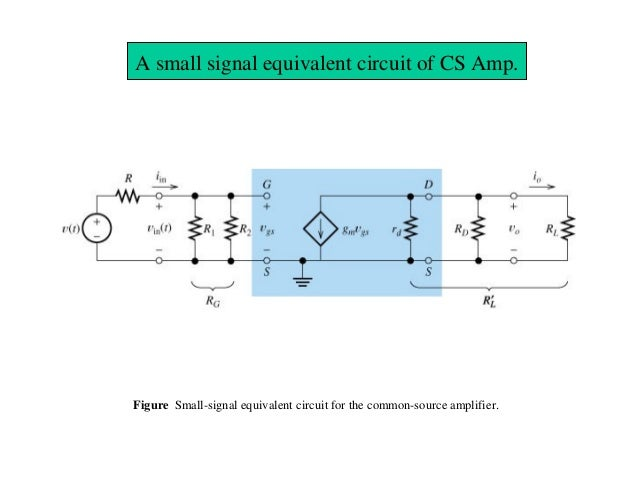# Common Source JFET small signal equivalent circuit

This circuit is equivalent to small-signal common source JFET amplifier:If you look at the input and output impedance you can see that Zin = R1 || R2 and Zout = rd || Rd || Rl. It can be seen from the small-signal model.

But shouldn't small-signal model look like this?I just want to understand these models and maybe derivate input and output impedance myself.

The ideal DC voltage source has 0Ω internal resistance. And that's why AC-signals are shorted by DC voltage source. DC voltage is always constant so for any change in current there is no change in the voltage. So there is 0 Ohm internal resistance. Additional in real life circuit we always uses a bypass capacitor connect parallel to DC Voltage. And this capacitor will short all AC-signal to the ground.

For example if we have a 9V DC voltage source, now if we smoothly change the current that is draw from this DC voltage source, from 40mA to 20mV (we change the resistance from 225Ω to 450Ω). We create AC-current. But the DC voltage do not change (0Ω internal resistance). So the dynamic resistance of DC-voltage source is equal to rd = 0V/20mA = 0Ω And this is why we say that DC-Voltage is a short for an AC-signals.

• But we deal with ideal parameters only in theory. In practice the voltage source will have some resistance and therefore models like this one do not apply in practice - I mean, the formula for input/output resistance does not apply in practical situation. Or does it?
– Keno
Apr 9, 2017 at 16:50
• In normal situation we use bypass capacitor across supply rails to reduce (bypass/short) the voltage source internal resistance. So do not worry abut this anymore
– G36
Apr 9, 2017 at 17:06
• Its only that it doesn't seems logical to me if I am considering the basic electronic principles... Looks like I'm not ready yet to understand all of this you were explaining about.
– Keno
Apr 9, 2017 at 20:07
• @Keno What thing is not logical to you? Can you elaborate?
– G36
Apr 10, 2017 at 3:31
• The thing that Vdd becomes ground in small signal models.
– Keno
Apr 10, 2017 at 17:32

DC sources are always grounded in small signal analysis and therefore you get R1 || R2.

• But why are they grounded? For simplicity of equation derivation of what?
– Keno
Apr 9, 2017 at 16:42
• Since you said DC sources are always grounded in small signal analysis, does that mean the Udd represents ground for AC signals?
– Keno
Apr 9, 2017 at 16:47
• Yes, Udd represents ground for AC signals.
– G36
Apr 10, 2017 at 18:57simulate this circuit – Schematic created using CircuitLab

• I'm a sincere admirer of your ability to use CircuitLab to draw almost everything. Apr 9, 2017 at 13:30
• Help me on this. How can these be made more clear? Apr 9, 2017 at 20:58